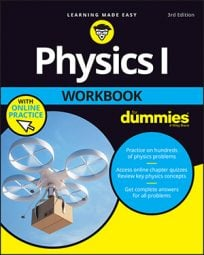##### Physics I Workbook For Dummies with Online PracticeThermodynamics is the study of how thermal energy (heat energy) and mechanical energy are related. It's an important topic in physics as well as in engineering. Engineers must employ thermodynamic principles whenever heat is involved. This includes the design of refrigerators, air conditioning units, automobiles, jet engines, and even computers.

One of the most important ideas within this topic is the first law of thermodynamics, which is a restatement of the law of conservation of energy, with an emphasis on the idea that mechanical energy and thermal energy can, indeed, be transformed into each other. It states that the change in energy of a gas is equal to the amount the gas is heated plus the amount of work that is done on that gas.

That's a mouthful, so the first law of thermodynamics is usually expressed as an equation:

U = Q + W

In this equation, ∆U is the change in internal energy of the gas (not including, for example, the energy due to the motion of the box containing the gas) and for an ideal gas is proportional to the change in temperature of the gas. Q is the amount the gas is heated, and W is the amount of work done on the gas. If the gas heats its surroundings (instead of the surroundings heating the gas), then Q is negative. Likewise, if the gas does work on its surroundings, then W is negative.

Here's a conceptual example: Imagine a balloon filled with helium. What happens to the balloon's temperature if you squeeze it? When you squeeze a balloon, you apply forces to that balloon's surface through a distance. In other words, you do work on the balloon. From the first law of thermodynamics, this means that you increase the internal energy, and thus the temperature, inside the balloon. The balloon's temperature then decreases again as the balloon heats its surroundings (a result of the second law of thermodynamics).

This example may seem trivial, but if you understand it, you're well on your way to understanding the first law of thermodynamics, as well as thermodynamics in general. In addition, this example is similar to what you find in everyday technology. Both refrigerators and air conditioning units contain compressors that act much as the balloon described.